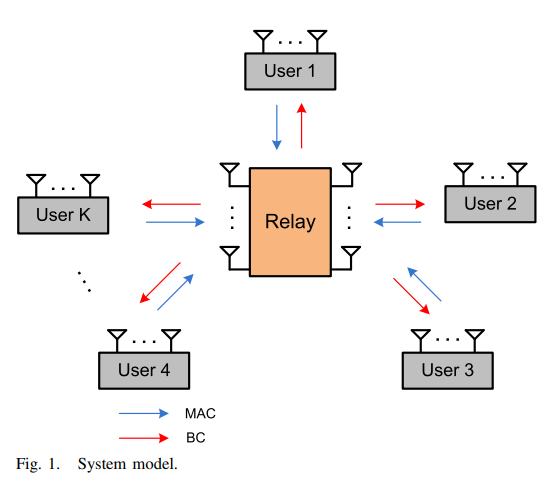Conference Paper

# Degrees of Freedom for the MIMO Multi-Way Relay Channel with Common and Private Messages

By
Salah M.
El-Keyi A.
Nafie M.
Mohasseb Y.

In this paper, we study the general multiple input multiple output (MIMO) multi-way relay channel, i.e., MIMO Y channel, with common and private messages. In this channel, K users exchange messages through a common relay. Each user transmits a private message to each user in addition to a common message to all the other users. The i th user and the relay are equipped with Mi and N antennas, respectively. First, we derive the degrees of freedom (DoF) region of the symmetric three-user MIMO Y channel, where Mi = M and i ∈ {1,2,3}. Due to the symmetry of the network, we focus on the case where the DoF of all private messages are equal and the DoF of all common messages are equal. In this case, the DoF region has two dimensions: the DoF of private messages and the DoF of common messages. We develop an outer bound on the DoF region based by using cut-set and one-sided genie bounds. We prove the achievability of the outer bound on the DoF region by using linear beamforming and signal space alignment (SSA) schemes. Second, based on our study of the DoF region of the symmetric channel, we define a weighted sum DoF metric that integrates all the network messages and weights the common messages by a factor of α. We study the weighted sum DoF maximization problem and show that sending common messages only is optimal when α exceeds 4/3. Next, we focus on the weighted sum DoF with α = 2 that represents the total number of received interference-free streams at the users. First, we show that the weighted sum DoF, with α = 2 , of the MIMO Y channel with an arbitrary number of antennas is given by min {3N,2N+M3,N+M2+M3,2M2+2M3, M1+M2+M3}. Second, we study the weighted sum DoF, with α = 2 , of the K -user case in the symmetric setting. We derive an outer bound on the weighted sum DoF using cut-set bounds, and show that the network has K min {N,M} weighted DoF. The achievability results are obtained by using SSA for network coding in the multiple access phase, and zero-forcing precoding in the broadcast phase. © 2016 IEEE.